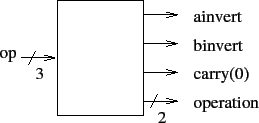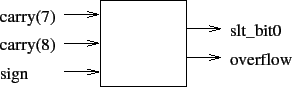# Homework VI

Tom Kelliher, CS 240

25 points, due April. 6

For this assignment, you will be implementing in VHDL and simulating an eight-bit version of the simplified arithmetic logic unit (ALU) used in Patterson & Hennessy's Computer Organization & Design textbook. Use the following for the top-level entity I/O structure of your ALU:

```entity alu8 is
Port ( a        : in   STD_LOGIC_VECTOR (7 downto 0);
b        : in   STD_LOGIC_VECTOR (7 downto 0);
op       : in   STD_LOGIC_VECTOR (2 downto 0);
result   : out  STD_LOGIC_VECTOR (7 downto 0);
overflow : out  STD_LOGIC
);
end alu;
```
The functions to be computed by the ALU are defined by:
```if (op == 0)
result = a AND b;
else if (op == 1)
result = a OR b;
else if (op == 2)
result = a NAND b;
else if (op == 3)
result = a NOR b;
else if (op == 4)
{
result = a + b;
if (overflow has occurred)
overflow = 1;
else
overflow = 0;
}
else if (op == 5)
{
result = a - b;
if (overflow has occurred)
overflow = 1;
else
overflow = 0;
}
else if (op == 6)   // set on less than AKA slt
if (a < b)
result = 1;
else
result = 0;
```
For those ops for which `overflow` is not defined, you may assume that it is a don't care. (Don't read too much into this -- it simply means that you only need be concerned with overflow's value being correct for the addition and subtraction operations.) You may assume that op's value will never be 7.

The eight-bit ALU just described can be broken down into eight one-bit ALUs and some combinational control logic. Use this entity statement for the one-bit ALU:

```entity alu1 is
Port ( a         : in  STD_LOGIC;
b         : in  STD_LOGIC;
less      : in  STD_LOGIC;
ainvert   : in  STD_LOGIC;
binvert   : in  STD_LOGIC;
carryin   : in  STD_LOGIC;
operation : in  STD_LOGIC_VECTOR (1 downto 0);
result    : out STD_LOGIC;
carryout  : out STD_LOGIC;
sign      : out STD_LOGIC
);
```
The following block diagram illustrates nearly all of the functionality required of the one-bit ALU. The one missing piece is the sign output defined in the above entity statement. Use the sum output directly from the one-bit full adder to produce the sign bit.Two small pieces of combinational logic are necessary. The first of these generates the control signals needed by the one-bit ALUs from the `op` input of the eight-bit ALU:The values of these output signals, needed by the one-bit ALUs, are completely determined by the value of `op`. For example, if `op` is `011` (NOR), then both `ainvert` and `binvert` should be `1`, `carry(0)` is a don't care, and `operation` should be `00`, selecting the value of the AND gate as the output of the mux.

The second piece of combinational logic is used to generate bit 0 of `result` for the `slt` instruction and to generate the `overflow` output:Some hints:

1. The simplest way of recognizing when overflow has occurred is by comparing the carryouts from the two most significant bits of the eight-bit ALU (bits 7 and 6). If they differ, overflow has occurred; otherwise, there is no overflow.

2. Recall that VHDL has an addition operator. Hence, there is no need for you to implement a one-bit full adder.

3. Within the eight-bit ALU, the sign bit (bit 7) is used as bit 0 of `result` for the `slt` instruction.

4. The `slt` instruction should work correctly for all values of `a` and `b`, even if overflow occurs. Remember, overflow indicates that the sign bit is wrong.

5. The 2-1 muxes and inverters used to conditionally invert `a` and `b` within the one-bit ALU can be replaced with fan-in 2 EXOR gates. (VHDL has an `xor` operator.)

6. The `sign` outputs of the seven least significant one-bit ALUs will be unneeded in the eight-bit ALU. You may use the `open` keyword in the port map lists for those seven ALUs to indicate that the sign output should be left unconnected.

Turn in your VHDL source code (module files and test bench files), your test waveforms from ISim, and a brief commentary defending your choice of test bench test cases as demonstrating the correctness of your eight-bit ALU.

Thomas P. Kelliher 2012-03-29
Tom Kelliher